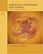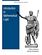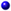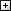Processing ......FreeComputerBooks.com Free Computer, Mathematics, Technical Books and Lecture Notes, etc.

Selected New Books in December, 2017
For Weather, Flights, Runways of Airports all over the world, etc, click here.
•Network Programming with Go (Jan Newmarch)

Post under Network Programming and Go Programming on Sat Dec 09, 2017

Dive into key topics in network architecture and Go, such as data serialization, application level protocols, character sets and encodings. This book covers network architecture and gives an overview of the Go language as a primer.

•Python Scientific Lecture Notes (Scipy Lectures )

Post under Python Programming on Sat Dec 09, 2017

This book is the teaching material on the scientific Python ecosystem, a quick introduction to central tools and techniques, for programmers from beginner to expert. Work on real-world problems with SciPy, NumPy, Pandas, scikit-image, and other libs.

•Modeling and Simulation in Python (Allen B. Downey)

Post under Computational Simulations & Modeling and Python on Sat Dec 09, 2017

This book is an introduction to physical modeling using a computational approach with Python. You will learn how to use Python to accomplish many common scientific computing tasks: importing, exporting, and visualizing data; numerical analysis; etc.

•A Friendly Introduction to Mathematical Logic (Chris Leary)

Post under Set Theory, Model Theory, Computability, etc on Sat Dec 09, 2017

In this user-friendly book, readers with no previous study in the field are introduced to the basics of model theory, proof theory, and computability theory, leading to rigorous proofs of Gödel's First and Second Incompleteness Theorems.

•The Little Book of Semaphores, Second Edition (Allen B. Downey)

Post under Concurrent Programming and Operating Systems on Sat Dec 09, 2017

This book introduces the principles of synchronization for concurrent programming. The approach of this book is to identify patterns that are useful for a variety of synchronization problems and then show how they can be assembled into solutions.

•Support Vector Machines Succinctly (Alexandre Kowalczyk)

Post under Machine Learning on Sat Dec 09, 2017

This book guides readers through the building blocks of Support Vector Machines (SVMs), from basic concepts to crucial problem-solving algorithms. It also includes numerous code examples and a lengthy bibliography for further study.

•Android Developer Fundamentals Course: Practical Workbook

Post under Android Development and Programming on Sat Dec 09, 2017

It prepares you to take the exam for the Associate Android Developer Certification. You learn basic Android programming concepts and build a variety of apps, starting with Hello World and working your way up to apps that use content providers and loaders.

•Linear Algebra: A Course for Physicists and Engineers (A. Mathai)

Post under Linear Algebra and Applied Mathematics on Sat Dec 09, 2017

This textbook on linear algebra is written to be easy to digest by non-mathematicians. It introduces the concepts of vector spaces and mappings between them without too much theorems and proofs. Various applications of the formal theory are discussed as well.

•Discrete Mathematics: An Open Introduction (Oscar Levin)

Post under Discrete and Finite Mathematics on Sat Dec 02, 2017

This is a gentle introduction to discrete mathematics. Four main topics are covered: counting, sequences, logic, and graph theory. Along the way proofs are introduced, including proofs by contradiction, proofs by induction, and combinatorial proofs.

•Modeling, Functions, and Graphs: Algebra for College Students

Post under Algebra, Abstract Algebra, and Linear Algebra on Sat Dec 02, 2017

This book includes content found in a typical algebra course, along with introductions to curve-fitting and display of data. The authors focus on three core themes throughout their textbook: Modeling, Functions, and Graphs.

•Elementary College Geometry (Henry Africk)

Post under Geometry and Algebra on Sat Dec 02, 2017

This text is intended for a brief introductory course in plane geometry, covers the topics from elementary geometry that are most likely to be required for more advanced mathematics courses. The only prerequisite is a semester of algebra.

•Introduction to Mathematical Logic (Vilnis Detlovs, et al)

Post under Set Theory, Model Theory, Computability, etc on Sat Dec 02, 2017

This book explores the principal topics of mathematical logic. It covers propositional logic, first-order logic, first-order number theory, axiomatic set theory, and the theory of computability. Discusses the major results of Gödel, Church, Kleene, Rosser, and Turing.

•Advances in Discrete Differential Geometry (Alexander I. Bobenko)

Post under Geometry and Discrete Mathematics on Sat Dec 02, 2017

It surveys the fascinating connections between discrete models in differential geometry and complex analysis, integrable systems and applications in computer graphics and is illustrated to convince readers it's both beautiful and useful.

Book CategoriesAll CategoriesRecent BooksIT Research LibraryMiscellaneous BooksComputer LanguagesComputer ScienceData Science/DatabasesElectronic EngineeringJava and Java EE (J2EE)Linux and UnixMathematicsMicrosoft and .NETMobile ComputingNetworking and CommunicationsSoftware EngineeringSpecial TopicsWeb Programming
Other Categories